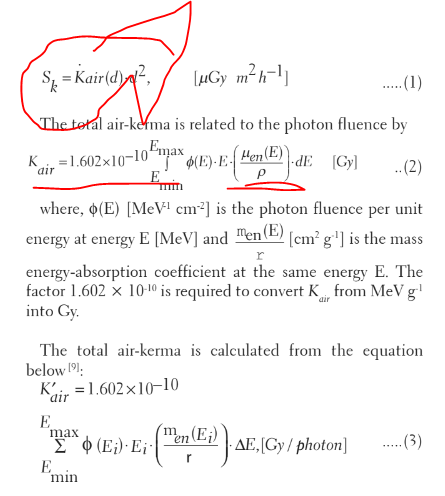# Dose scoring and calculations

Dear Geant4 experts would give me some idea about … In the advanced examples there is a brachytherapy example. It is used different radionuclide sources to calculate the dose or to score the dose. Here I need to calculate air karma strength of one source(Ks). In general the equation is given as in the snapshot below. Here is it possible to score the air karma but what I didn`t understand is whether the constant 1.602*10^-10 is including in the Geant4 cross section or how I can include in my calculation. This question may be for those who prepared Advanced brachy therapy example. Thank you for your reply